# A* Search

A* Search is an informed best-first search algorithm that efficiently determines the lowest cost path between any two nodes in a directed weighted graph with non-negative edge weights. This algorithm is a variant of Dijkstra’s algorithm. A slight difference arises from the fact that an evaluation function is used to determine which node to explore next.

## Evaluation Function

The evaluation function, f(x), for the A* search algorithm is the following:

``````f(x) = g(x) + h(x)
``````

Where g(x) represents the cost to get to node x and h(x) represents the estimated cost to arrive at the goal node from node x.

For the algorithm to generate the correct result, the evaluation function must be admissible, meaning that it never overestimates the cost to arrive at the goal node.

## The Algorithm

The A* algorithm is implemented in a similar way to Dijkstra’s algorithm. Given a weighted graph with non-negative edge weights, to find the lowest-cost path from a start node S to a goal node G, two lists are used:

• An open list, implemented as a priority queue, which stores the next nodes to be explored. Because this is a priority queue, the most promising candidate node (the one with the lowest value from the evaluation function) is always at the top. Initially, the only node in this list is the start node S.
• A closed list which stores the nodes that have already been evaluated. When a node is in the closed list, it means that the lowest-cost path to that node has been found.

To find the lowest cost path, a search tree is constructed in the following way:

1. Initialize a tree with the root node being the start node S.
2. Remove the top node from the open list for exploration.
3. Add the current node to the closed list.
4. Add all nodes that have an incoming edge from the current node as child nodes in the tree.
5. Update the lowest cost to reach the child node.
6. Compute the evaluation function for every child node and add them to the open list.

Just like in Dijkstra’s algorithm, the lowest cost is updated as the algorithm progresses in the following way:

``````current_lowest_cost = min(current_lowest_cost, parent_node_cost + edge_weight)
``````

All nodes except for the start node start with a lowest cost of infinity. The start node has an initial lowest cost of 0.

The algorithm concludes when the goal node G is removed from the open list and explored, indicating that a shortest path has been found. The shortest path is the path from the start node S to the goal node G in the tree.

Note: The algorithm ends when the goal node G has been explored, NOT when it is added to the open list.

## Example

Consider the following example of trying to find the shortest path from S to G in the following graph: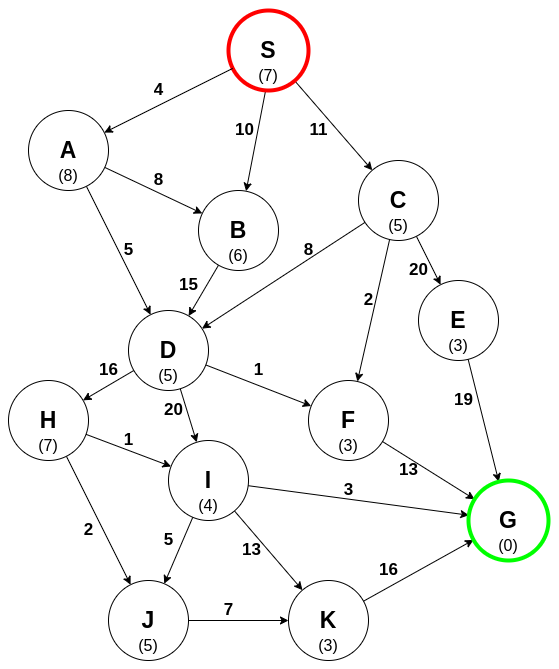Each edge has an associated weight, and each node has a heuristic cost (in parentheses).

An open list is maintained in which the node S is the only node in the list. The search tree can now be constructed.

Exploring S: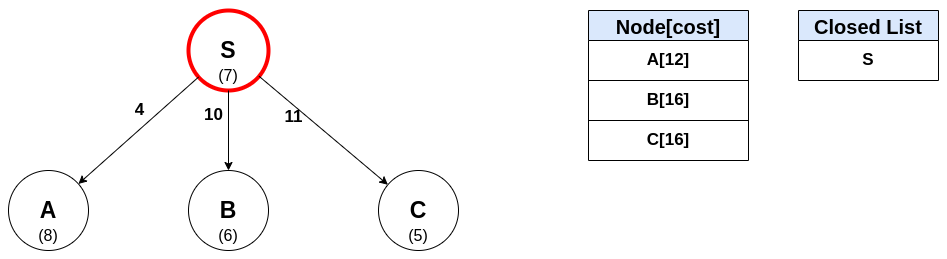A is the current most promising path, so it is explored next: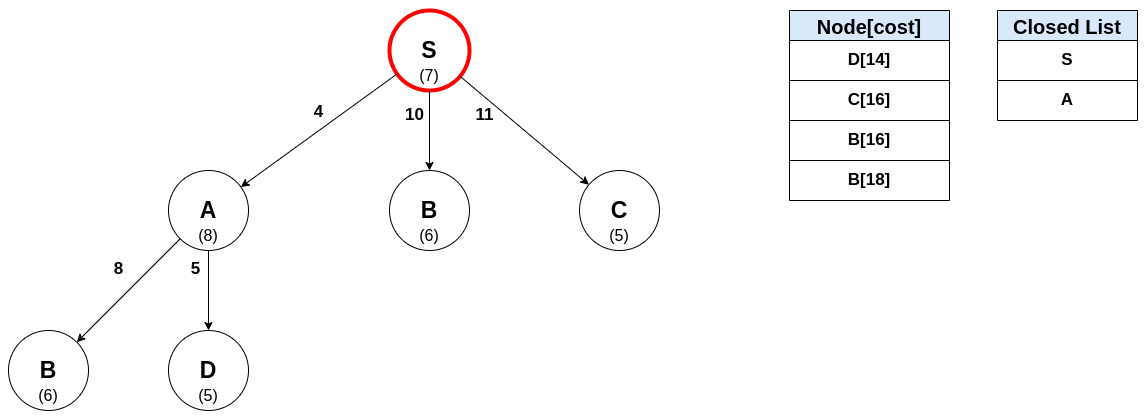Exploring D: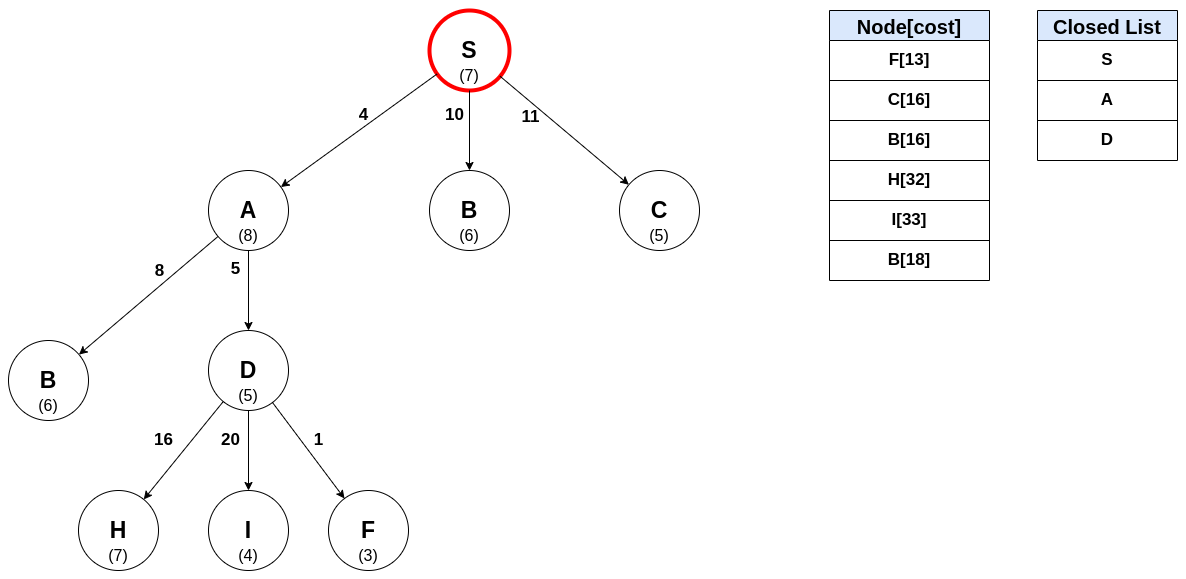Exploring F: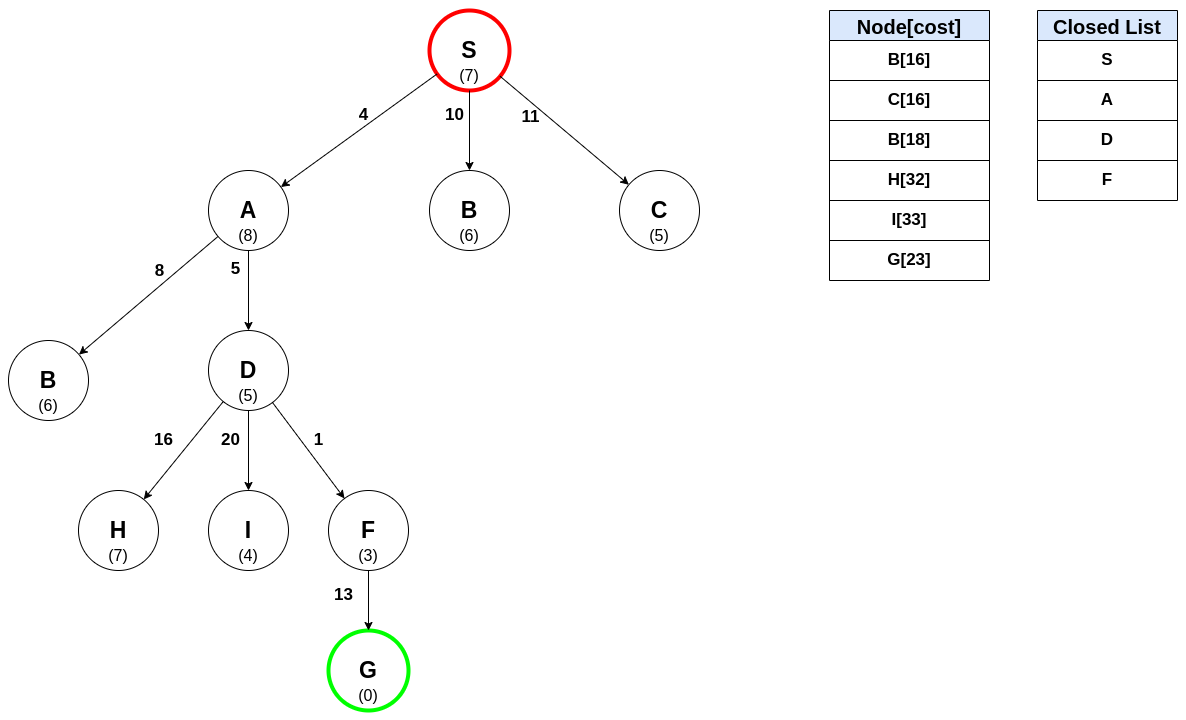Notice that the goal node G has been found. However, it hasn’t been explored, so the algorithm continues because there may be a shorter path to G. The node B has two entries in the open list: one at a cost of 16 (child of S) and one at a cost of 18 (child of A). The one with the lowest cost is explored next: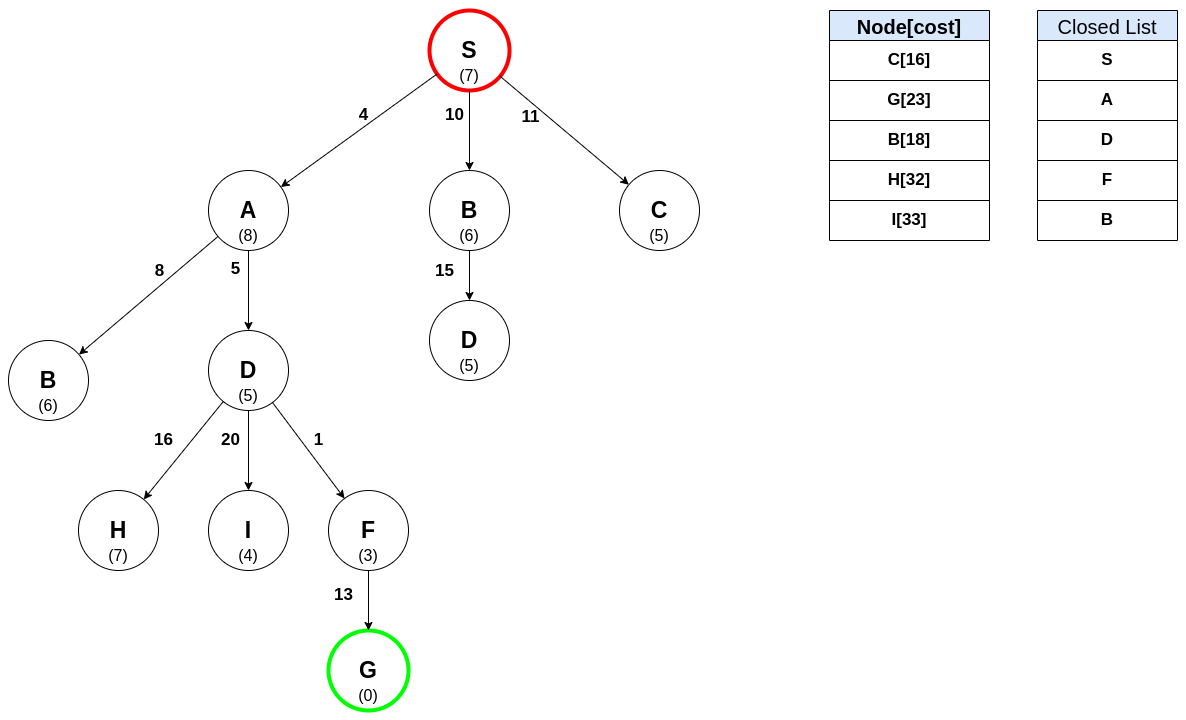Exploring C: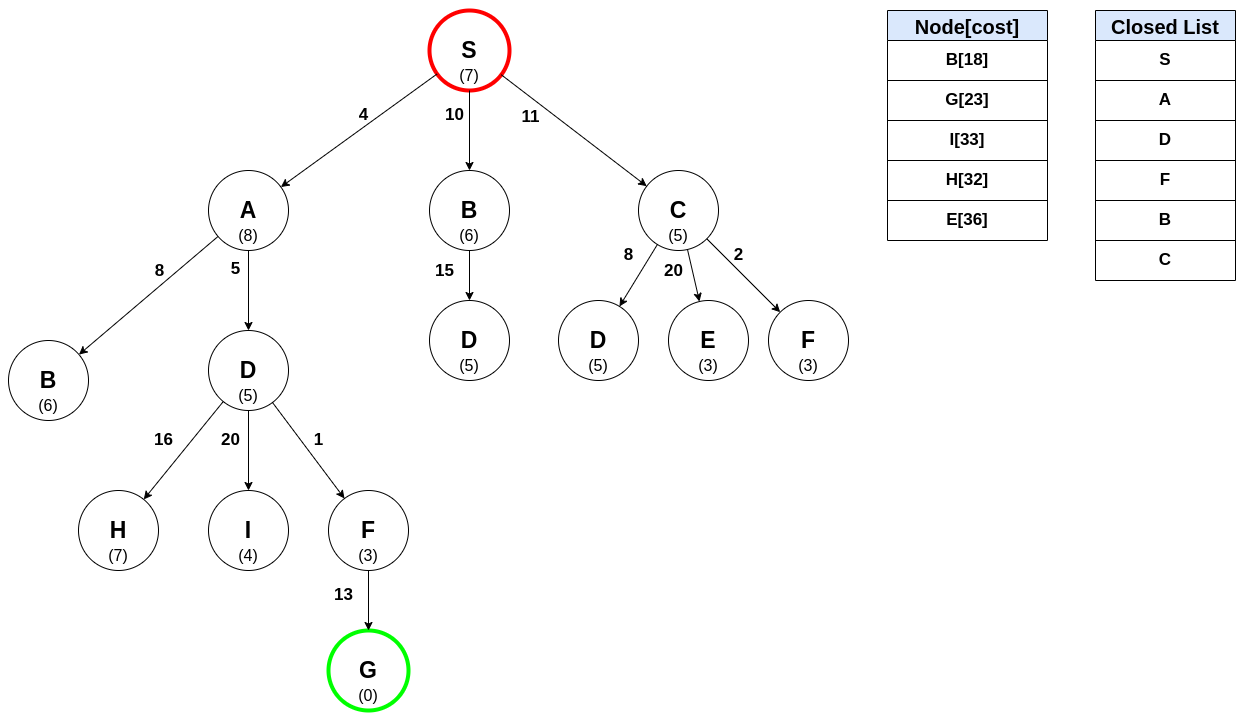The next node in the open list is again B. However, because B has already been explored, meaning a shortest path to B has been found, it is not explored again and the algorithm continues to the next candidate.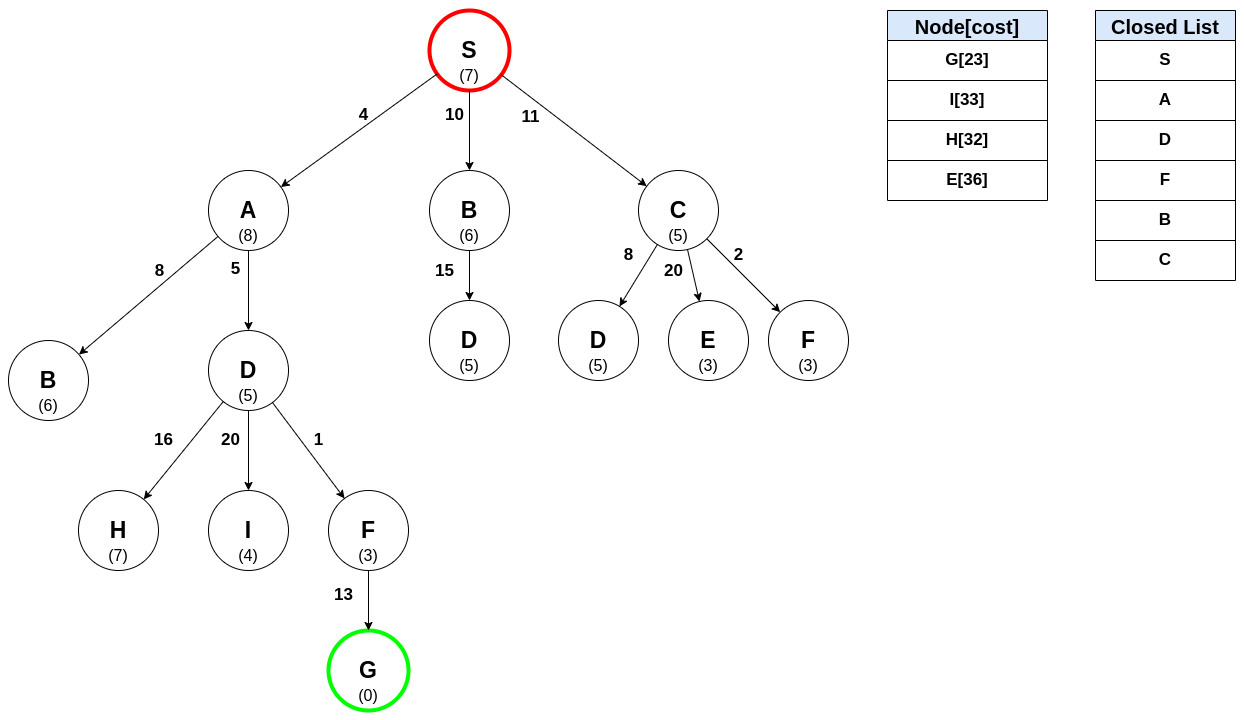The next node to be explored is the goal node G, meaning the shortest path to G has been found! The path is constructed by tracing the graph backward from G to S: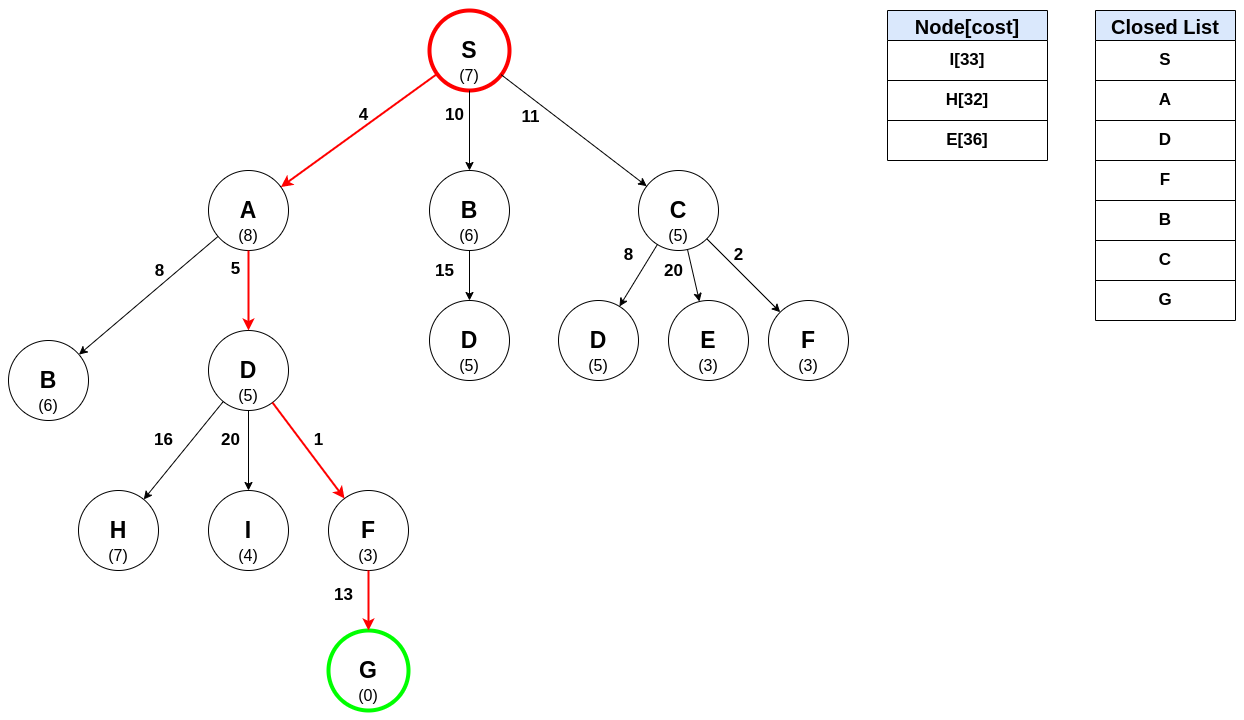## Using the A* Algorithm

This algorithm is guaranteed to find a shortest path if one exists. One of the main uses of this algorithm is route planning. However, there are many other uses.